RERTR Reduced Enrichment for Research and Test Reactors Nuclear Science and Engineering Division at ArgonneHome  :: Foreign Research Reactor Spent Nuclear Fuel :: Related Documents

### RERTR Publications: Foreign Research Reactor Spent Nuclear Fuel

#### ANL/RERTR/TM-26

##### THERMAL DECAY HEAT

The textbook development of calculating the thermal decay heat of reactor fuel is based upon integrating empirical emission rates of the beta and gamma radiation from fission products. These results are, however, generally useful only for fission product decay times of the order of a few days. For longer decay times, this heat load estimate is very conservative.

Other analytical expressions have been developed to fit experimental decay heat data for longer decay times. For purposes of determining the heat load of spent fuel which can have cooling times of the order of several hundred days of even years, these latter expressions should be used to calculate the heat load of spent reactor fuel. These analytical expressions also agree very well with ORIGEN decay heat calculations.

Integrated Beta And Gamma Emission Rates

The heat load from decaying fission products in a fuel assembly is proportional to empirical emission rates of beta and gamma radiation. The rates4 per U-235 fission, and as a function of decay time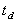in days, are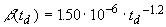MeV/s-f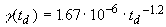MeV/s-f

These energy rates are roughly equal for 0.4 MeV mean energy beta particles and 0.7 MeV mean energy gamma-rays.

For a fuel assembly irradiated continuously for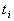days at a constant fuel assembly power (P), the heat (H) load power per assembly,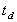days after irradiation isWatts (1)

This expression for the heat load is the integral5 of the above energy rates over the irradiation time, assuming 200 MeV per U-235 fission, and for the fuel assembly power in watts. For a low duty-factor fuel assembly irradiation, the power and irradiation time are replaced by an average power and an elapsed time. With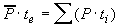over all irradiation segments, the heat (H) load power per assembly is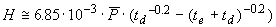Watts

whereis the average fuel assembly power in watts and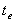is the elapsed time in days from the initial through the final irradiation segment.

A convenient estimate for the average power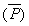is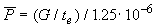Watts

where G is the mass of U-235 burned in the fuel assembly in grams, and the constant is g235U burned per Watt-day.

A similar heat load expression to Eq. -1, given by Etherington (Ref. 6) and attributed to Way and Wigner (Ref. 7 and 8), is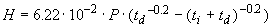Watts

with all times in seconds. With all times in days, this heat load expression is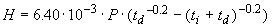Watts

(Note, the Etherington reference to Way and Wigner appears to be incorrect. Reference 7 is Vol. 73 (not Vol. 70)6 of Phys. Rev.; Ref. 8 may be the intended reference. However neither Ref. 7 or 8 appears to have the formula attributed to Way and Wigner. Reference 8 however, lists the same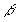and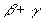emission rates used to develop Eq. -1 which results in a heat load expression constant of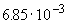.) This and other similar heat load expressions, which differ only by the constant, can be readily found in the literature (e.g., Ref. 5,for times in seconds and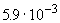for times in days).

Fuel assembly decay heat loads calculated with these expressions are expected to be conservative, and within a factor of two or less of measured heat loads. This same conservative heat load estimate also has been found to be true for heat load calculations made with the ORIGEN code9. The thermal heat load of a fuel assembly is independent of the fuel assembly type.

The constants used in the above equations are based upon empirical data and therefore, are not necessarily exact; it is not uncommon to find several percent variation in a recommended value. The constants considered here, and their range, are:

1. the beta plus gamma fission product energy rate per fission;

2.7 - 3.2 ·10-6 MeV/s-f,

1. the total energy release per fission; 190 - 200 MeV/f, and
2. the mass of 235U burned per megawatt-day; 1.2 - 1.3 g235U/MWd.

Depending upon the specific values of the constants that are chosen, the calculated heat load can vary by several percent. In any case, the thermal decay heat is expected to be over predicted.

Decay Heat Curves

An analytical expression given by El-Wakil (Ref. 10), which correlate with the decay heat curves of Ref. 11, estimate heat loads about one-half the heat loads calculated above. This heat load expression is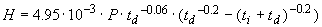Watts (2)

where all symbols, etc. have the same meaning as above and the times are in days.

The ratio of Eq. -2 to Eq. -1 is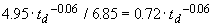For decay times (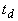) greater than 1 year, the ratio is approximately 0.5.

Experimental Decay Heat Data

Another analytical expression given by Untermyer and Weills (Ref. 12), has been used to fit experimental decay heat data. This heat load expression is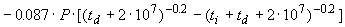Watts (3)

where the irradiation () and decay (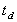) times are in seconds.

A plot of the ratio (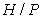) for Eqs. -2 and -3 are shown in Fig. 2 as a function of decay time and for an irradiation time of 600 days. The ratio calculated with the ORIGEN code is also shown for comparison.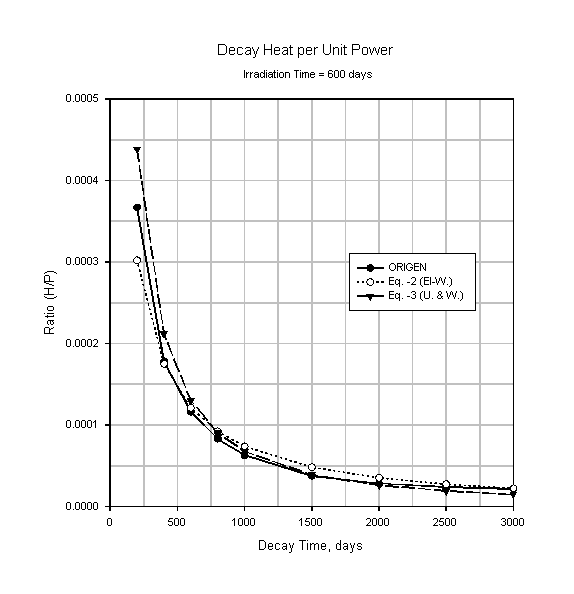Figure 2. Comparison of Decay Heat Equations-2 and -3 with ORIGEN

These data clearly show the relative decay heat estimated by the decay heat expressions for a typical irradiation time. The ORIGEN ratio is in good agreement with both Eqs. -2 and -3.The 2016 International RERTR Meeting (RERTR-2016) will take place in Belgium. Stay tuned for further details. The 2015 International RERTR Meeting (RERTR-2015) took place in Seoul, Korea on Oct. 11-14, 2015. For more information visit RERTR-2015.ANL/RERTR/TM-26Introduction Nuclear Mass Inventory Photon Dose Rate Thermal Decay Heat Conclusions References Appendix A: MTR Model Mass Inventory Sensitivity Appendix B: U-234 And U-236 Mass Inventory Sensitivity Appendix C: Mass Inventory Estimate: ORIGEN Vs. WIMS Appendix D: Example Calculation: Nuclear Mass Inventory, Photon Dose Rate And Thermal Decay HeatPDF Version the full paper in PDF formatARGONNE NATIONAL LABORATORY, Nuclear Engineering Division, RERTR Department 9700 South Cass Ave., Argonne, IL 60439-4814 A U.S. Department of Energy laboratory managed by UChicago Argonne, LLCLast modified on July 29, 2008 11:34 +0200